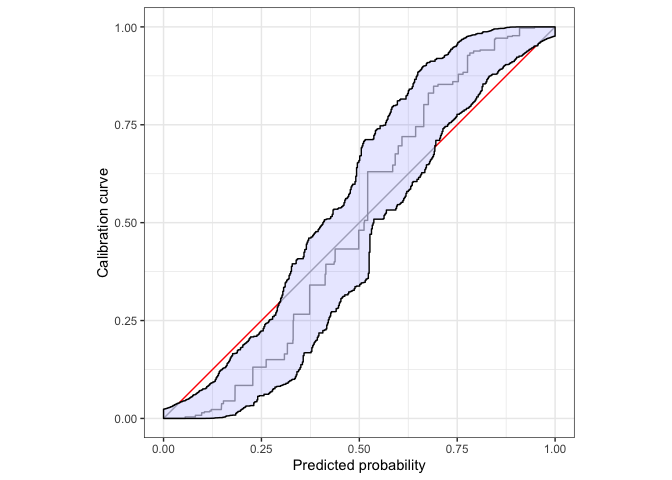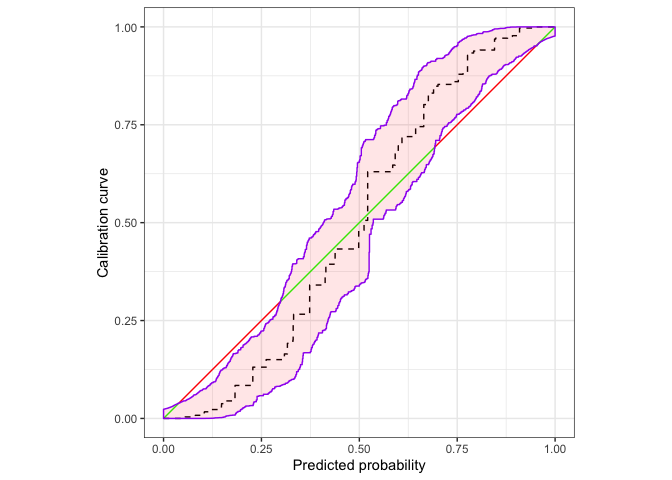An R package to assess calibration of binary outcome predictions. Authored by Timo Dimitriadis (Heidelberg University), Alexander Henzi (University of Bern), and Marius Puke (University of Hohenheim).

## Installation

calibrationband is available on The Comprehensive R Archive Network (CRAN).

install.packages("calibrationband")

### Development version

The most current version is available from GitHub.

# install.packages("devtools")
devtools::install_github("marius-cp/calibrationband")

## Example

library(calibrationband)
library(dplyr)
set.seed(123)
s=.8
n=10000
x <- runif(n)
p <- function(x,s){p = 1/(1+((1/x*(1-x))^(s+1)));return(p)}
dat <- tibble::tibble(pr=x, s=s, cep = p(pr,s), y=rbinom(n,1,cep))%>% dplyr::arrange(pr)

cb <- calibration_bands(x=dat$pr, y=dat$y,alpha=0.05, method = "round", digits = 3)
print(cb) # prints autoplot and summary, see also autoplot(.) and summary(.)#>
#>  Areas of misscalibration (ordered by length). In addition there are 2 more.
#> # A tibble: 5 × 2
#>    min_x  max_x
#>    <dbl>  <dbl>
#> 1 0.0396 0.299
#> 2 0.693  0.951
#> 3 0.0389 0.0393
#> # … with 2 more rows

Use ggplot2:autolayer to customize the plot.

autoplot(cb,approx.equi=500, cut.bands = F,p_isoreg = NA,p_ribbon = NA,p_diag = NA)+
ggplot2::autolayer(
cb,
cut.bands = F,
p_diag = list(low = "green", high = "red", guide = "none", limits=c(0,1)),
p_isoreg = list(linetype = "dashed"),
p_ribbon = list(alpha = .1, fill = "red", colour = "purple")
)